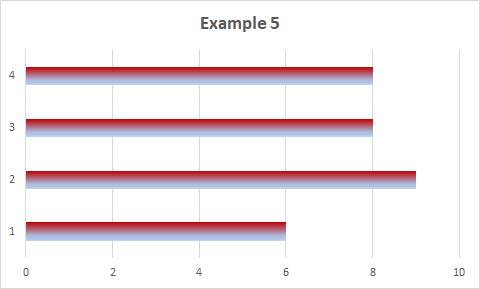## 17 October, 2021

### TOP TIP: Counting Cells Containing Numbers Meeting Specific Criteria

Most of the time we organize our data in orderly Excel tables making their analysis pretty straightforward. Sometimes, however, we may have to deal with chaotic sets of numbers located all over different ranges/worksheets, like in this somewhat bloated example:

How to count and analyse them? Couple of examples follow.

Example 1

For basic counting of all cells containing numbers within a selected range(s) we can use a simple formula, e.g.:

=COUNT(B2:F10)

What if we want to get some idea about e.g. distribution of those numbers, or we'd like to find out if there are any outliers in the set of data, etc.. Among many possible solutions, I'd recommend using just one amazing ARRAY FORMULA, in many available formats. Here are some some of them.

Example 2

Having counted all cells containing numbers, now you want to know how many of those numbers are higher than 50:

=INDEX(FREQUENCY((B2:F10),50),2)

or equal to and lower than 50:

=INDEX(FREQUENCY((B2:F10),50),1)

Example 3

Your data are located in three different ranges and you'd like to know how many of the numbers exceed 22:

=INDEX(FREQUENCY((A4:A10,B3:C21,R8:R25),22),2)

Those three array formulas shown above are SINGLE-CELL arrays. After typing them in, you just press CTRL+SHIFT+ENTER keys to enter them.

Example 4

You want to know distribution of the numbers within selected range in these 3 intervals, .e.g.: <=5, >5 and <=20, >20

=INDEX(FREQUENCY((B2:F10),{5,20}),{1;2;3})

This is MULTI-CELL array formula. To enter this array - first select three cells in a column, and then type the formula in and press CTRL+SHIFT+ENTER keys.

Example 5

You want to know distribution of the numbers within selected range in these 4 intervals, e.g.: <=4, >4 and <=9, >9 and <=22, >22:

=INDEX(FREQUENCY((B2:F10),{4,9,22}),{1;2;3;4})

This is also MULTI-CELL array formula. To enter this array - first select four cells in a column, and then type the formula in and press CTRL+SHIFT+ENTER keys.

Here are the results of all those formulas:

Looking at consecutive formulas and the results displayed here you can see the logic behind them. In the last two examples the formulas use array constant as their last component (argument). You create the constants by entering a list of items within curly braces, {}. In the examples, the items are separated by semicolons, so the results are arranged vertically (in a column). If you separate the items using commas, the results will be arranged in a row (horizontally).

Following the examples, if you're dealing - in any real data analysis - with a high volume of numeric (or mixed) type of data, you can create similar array formulas with appropriate array constants to obtain distribution list with preselected boundaries (e.g. 1-10, 11-20, 21-30, 31-40...etc.), and use it in your analysis. If needed, the array constants can be entered in a worksheet cells and named, as any other range.

All can be done with just one easily editable formula that works with:

• numbers entered in any order and in discontinuous ranges (with some empty cells)
• numbers mixed with textual entries, and
• numbers resulting from regular Excel formulas

And the results of counting with array constants can be utilized e.g. for graphical presentation (charts). Check it for yourself.Important note:

Some dates are included on purpose (coloured differently) in my example of data range used for counting.

Remember that if there are cells formatted as DATE or TIME in the selected range of your data, they are treated as serial numbers and included in the counting. So, you might need to exclude them from the analysis. Otherwise they may distort the results.